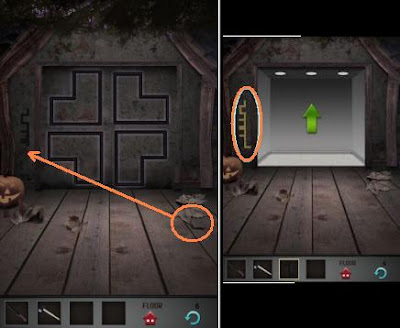# 100 Floors Seasons 7

After 6 stage passed most of game players stuck. how to pass 100 floors seasons 7 ? it's easy if you can break the code behind the number. follow our guide belowFirst let's begin the game with previous level : stage 6

• Get a pice of code under leafes, it's on the ground in the right side
• Then put that missing code to the place on wall in the left side of door
• Now follow the code. it's letter L and match it with L shape on the door
• TR-BL-BL-TL-TL-TR-TL
• (TL for top left , BL for bottom left, TR for top right)

100 Floors Seasons 7This is a math puzzle, Do you like math? don;t worry because you can beat this game with our hints below

• first look at the clue of the game : 0+4 = 8 and other clue is 2x7+8=20
• Do not look at the number but make it to the word and count the letter,
• 0 = zero = 4 letters
• 4 = four = 4 letters
• so 4+4=8

You can do the same to other equation in "100 floors seasons 7"
Then you can change this one 2+5+9x7+0x10=? to 3+4+4x5+4x3=?
Do multiplier first, so 3+4 + (4x5) + (4x3)=? it's 39
Now you can understand why 39 is the game answer

For Detail of 100 floors seasons 7 , please look at the picture here